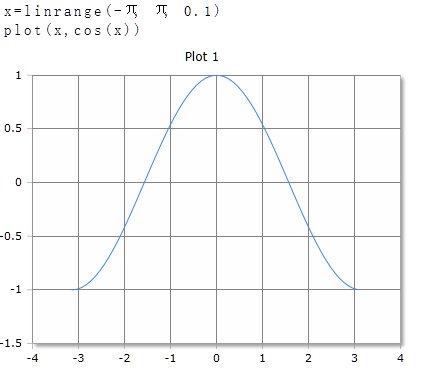# Cos Function

Returns the cosine of the argument

## Description

The Function $$Cos$$ returns the cosine of real or complex numbers. The argument can be a single number or a data field. For data fields, the cosine of each element is calculated and the results returned in a data field of equal size.

## Cos for real numbers

### Parameter

The angle is given in degrees (full circle = 360°) or radians (full circle = 2·p). The unit of measurement is set in the toolbar with the DEG or RAD buttons. The Setting applies to the entire worksheet.

### Result

Cos returns a value in the range of -1 to 1

### Optionaler Parameter

Optionally, a second parameter with the keywords DEG or RAD can be specified to set the unit of measure for this function call. The specification of the parameter has priority over the global setting in the toolbar. For different functions, you can use different units of measurement regardless of the default setting in the toolbar.

Cos (x)

Cos (x, deg)

### Example## Cos for complex numbers

For complex numbers, the argument and the result is always given as radian, regardless of the default setting in the toolbar. The result is also a complex number.

Cos(x + i)

### Example

Cos(2+3i)=-4.19-9.11i